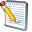## Project Properties – Calculations

The Calculations section configures how certain aspects of the application will calculate or display information within the project.

To change the information in this section, click the Edit buttonin the ribbon menu.Project Properties window – Calculations

#### RESOURCES

The Resource section lists options relating to calculations affecting the result of both Base and Group Resources.

OPTION

DESCRIPTION

Include Resource Units per Unit in Resource Unit Sum calculations

By default, the calculation and display of unit summations throughout the application do not include any resource units per unit specified on resources. This option will toggle whether Resource Units per Unit are included in calculations.

Genesis and Express editions only.

#### ESTIMATED COSTS

The Estimated Cost section allows you to configure how values within the project will be displayed.

OPTION

DESCRIPTION

Displayed Values of Unfinished Items

Enables unfinished Cost Schedule items to produce an Estimated Rate and Total. It also allows Group Resources to generate a Calculated Rate without needing to be finished.

Note: While a project contains unfinished items, spread values will not calculate.

#### MARGIN

The Margin section allows you to configure which values will be included (or excluded) when generating the margin to be applied.

OPTION

DESCRIPTION

Include Provisional Sum items when calculating ‘of Project Total’ margin

The total value of provisional sums used in the Cost Schedules will be included in only the calculation of overall or corporate margin types that have been set as ‘of Project Total’. This option is enabled by default.

#### OF PROJECT TOTAL MARGIN

This option allows you to set how Expert Estimation will calculate margin when both the Overall and Corporate Margins are set to the ‘of Project Total’ margin type.

OPTION

DESCRIPTION

Individually calculate Overall and Corporate Margins

The Overall and Corporate margins will calculate the ‘of Project Total’ margin exclusive of each other.

Example: Overall Margin is set to 15% of Project Total and Corporate Margin is set to 10% of Project Total.

The calculation will first use the 15% Overall Margin to calculate the first set of values, ignoring the 10% that would be created by Corporate Margin. Once the first calculation is complete, Expert Estimation will calculate the 10% Corporate Margin in a separate calculation ignoring any value generated by the Overall Margin.

Genesis and Express editions only.

Combine Overall and Corporate Margin Calculations

Both the Overall and Corporate Margin percentages will be combined to perform a single ‘of Project Total’ margin calculation.

Example: Overall Margin is set to 15% of Project Total and Corporate Margin is set to 10% of Project Total.

The calculation will combine the two percentages so that the ‘of Project Total’ calculation will use a percentage value of 25% when calculating the value of margin to generate. Once the margin value has been derived, it is then proportioned between the Overall and Corporate Margins.

Genesis and Express editions only.